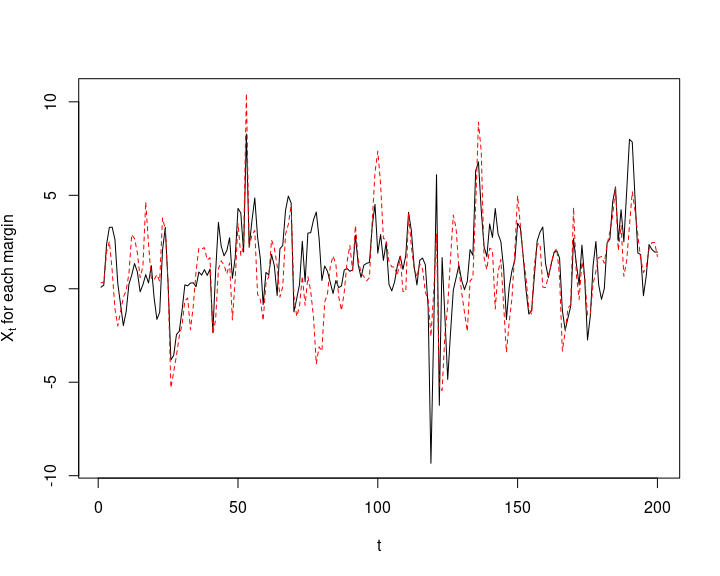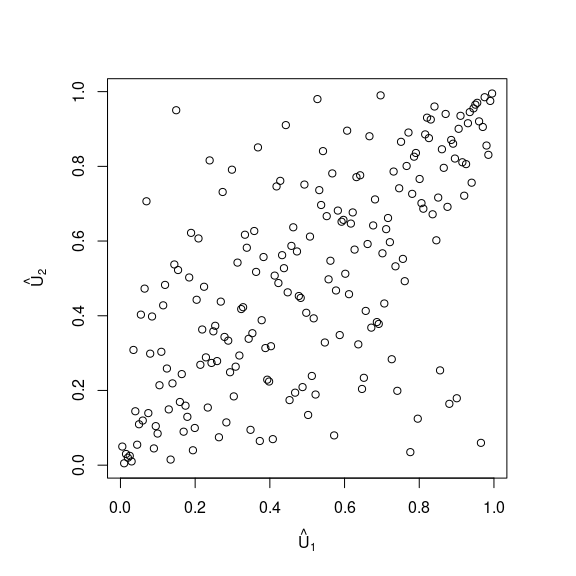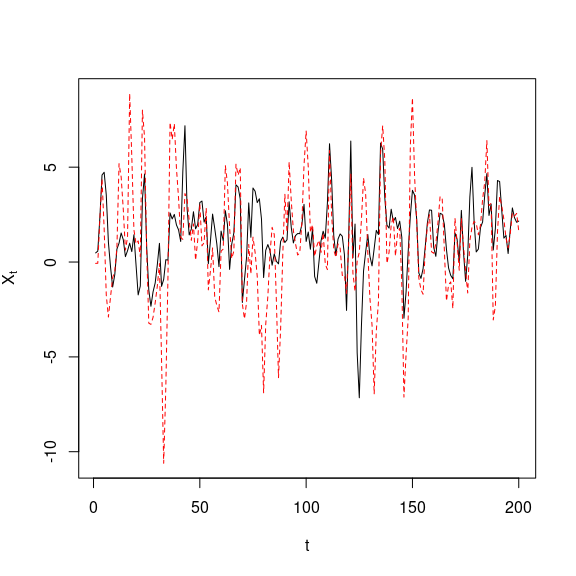# The Copula GARCH Model

#### 2019-04-21

require(copula)
require(rugarch)

In this vignette, we demonstrate the copula GARCH approach (in general). Note that a special case (with normal or student $$t$$ residuals) is also available in the rmgarch package (thanks to Alexios Ghalanos for pointing this out).

## 1 Simulate data

First, we simulate the innovation distribution. Note that, for demonstration purposes, we choose a small sample size. Ideally, the sample size should be larger to capture GARCH effects.

## Simulate innovations
n <- 200 # sample size
d <- 2 # dimension
nu <- 3 # degrees of freedom for t
tau <- 0.5 # Kendall's tau
th <- iTau(ellipCopula("t", df = nu), tau) # corresponding parameter
cop <- ellipCopula("t", param = th, dim = d, df = nu) # define copula object
set.seed(271) # reproducibility
U <- rCopula(n, cop) # sample the copula
nu. <- 3.5 # degrees of freedom for the t margins
Z <- sqrt((nu.-2)/nu.) * qt(U, df = nu.) # margins must have mean 0 and variance 1 for ugarchpath()!

Now we simulate two ARMA(1,1)-GARCH(1,1) processes with these copula-dependent innovations. To this end, recall that an ARMA($$p_1$$,$$q_1$$)-GARCH($$p_2$$,$$q_2$$) model is given by \begin{align} X_t &= \mu_t + \epsilon_t\ \text{for}\ \epsilon_t = \sigma_t Z_t,\\ \mu_t &= \mu + \sum_{k=1}^{p_1} \phi_k (X_{t-k}-\mu) + \sum_{k=1}^{q_1} \theta_k \epsilon_{t-k},\\ \sigma_t^2 &= \alpha_0 + \sum_{k=1}^{p_2} \alpha_k (X_{t-k}-\mu_{t-k})^2 + \sum_{k=1}^{q_2} \beta_k \sigma_{t-k}^2. \end{align}

## Fix parameters for the marginal models
fixed.p <- list(mu  = 1,
ar1 = 0.5,
ma1 = 0.3,
omega  = 2, # alpha_0 (conditional variance intercept)
alpha1 = 0.4,
beta1  = 0.2)
meanModel <- list(armaOrder = c(1,1))
varModel <- list(model = "sGARCH", garchOrder = c(1,1)) # standard GARCH
uspec <- ugarchspec(varModel, mean.model = meanModel,
fixed.pars = fixed.p) # conditional innovation density (or use, e.g., "std")

## Simulate ARMA-GARCH models using the dependent innovations
## Note: ugarchpath(): simulate from a spec; ugarchsim(): simulate from a fitted object
X <- ugarchpath(uspec,
n.sim = n, # simulated path length
m.sim = d, # number of paths to simulate
custom.dist = list(name = "sample", distfit = Z)) # passing (n, d)-matrix of *standardized* innovations

## Extract the resulting series
X. <- fitted(X) # X_t = mu_t + eps_t (simulated process)
sig.X <- sigma(X) # sigma_t (conditional standard deviations)
eps.X <- X@path$residSim # epsilon_t = sigma_t * Z_t (residuals) ## Basic sanity checks : stopifnot(all.equal(X., X@path$seriesSim, check.attributes = FALSE),
all.equal(sig.X, X@path$sigmaSim, check.attributes = FALSE), all.equal(eps.X, sig.X * Z, check.attributes = FALSE)) ## Plot (X_t) for each margin matplot(X., type = "l", xlab = "t", ylab = expression(X[t]~"for each margin"))## 2 Fitting procedure based on the simulated data We now show how to fit an ARMA(1,1)-GARCH(1,1) process to X (we remove the argument fixed.pars from the above specification for estimating these parameters): uspec <- ugarchspec(varModel, mean.model = meanModel, distribution.model = "std") fit <- apply(X., 2, function(x) ugarchfit(uspec, data = x)) Check the (standardized) Z, i.e., the pseudo-observations of the residuals Z: Z. <- sapply(fit, residuals, standardize = TRUE) U. <- pobs(Z.) par(pty = "s") plot(U., xlab = expression(hat(U)), ylab = expression(hat(U)))Fit a $$t$$ copula to the standardized residuals Z. For the marginals, we also assume $$t$$ distributions but with different degrees of freedom; for simplicity, the estimation is omitted here. fitcop <- fitCopula(ellipCopula("t", dim = 2), data = U., method = "mpl") ## Warning in var.mpl(copula, u): the covariance matrix of the parameter ## estimates is computed as if 'df.fixed = TRUE' with df = 2.3116814132331 nu. <- rep(nu., d) # marginal degrees of freedom; for simplicity using the known ones here est <- cbind(fitted = c(fitcop@estimate, nu.), true = c(th, nu, nu.)) # fitted vs true rownames(est) <- c("theta", "nu (copula)", paste0("nu (margin ",1:2,")")) est ## fitted true ## theta 0.6724201 0.7071068 ## nu (copula) 2.3116814 3.0000000 ## nu (margin 1) 3.5000000 3.5000000 ## nu (margin 2) 3.5000000 3.5000000 ## 3 Simulate from the fitted time series model Simulate from the fitted copula model. set.seed(271) # reproducibility U.. <- rCopula(n, fitcop@copula) Z.. <- sapply(1:d, function(j) sqrt((nu.[j]-2)/nu.[j]) * qt(U..[,j], df = nu.[j])) ## => Innovations have to be standardized for ugarchsim() sim <- lapply(1:d, function(j) ugarchsim(fit[[j]], n.sim = n, m.sim = 1, custom.dist = list(name = "sample", distfit = Z..[,j, drop = FALSE]))) and plot the resulting series ($$X_t$$) for each margin X.. <- sapply(sim, function(x) fitted(x)) # simulated series X_t (= x@simulation$seriesSim)
matplot(X.., type = "l", xlab = "t", ylab = expression(X[t]))